Ch 12. Infinite Sequences and Series Multimedia Engineering Math Sequencesand Series Integral andComparison Alt. Series &Abs. Conv. PowerSeries Taylor, Mac. &Binomial
 Chapter 1. Limits 2. Derivatives I 3. Derivatives II 4. Mean Value 5. Curve Sketching 6. Integrals 7. Inverse Functions 8. Integration Tech. 9. Integrate App. 10. Parametric Eqs. 11. Polar Coord. 12. Series Appendix Basic Math Units Search eBooks Dynamics Fluids Math Mechanics Statics Thermodynamics Author(s): Hengzhong Wen Chean Chin Ngo Meirong Huang Kurt Gramoll ©Kurt GramollMATHEMATICS - THEORY Power series, which are encountered often in science and engineering applications, will be presented in this section. An important function known as the Bessel function will also be discussed briefly. Power Series A series of the following form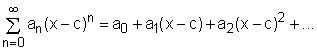is referred to as a power series about c or a power series centered at c. x is the variable and an's are the coefficients of the series. The sum of a power series can be represented using a function: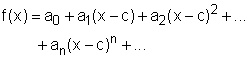where the function f(x) resembles a polynomial. A power series can either converge or diverge for a given value of x. One can use the convergence testing methods introduced previously (e.g., ratio test and root test) to determine if a power series is convergent or divergent. The following theorem can also be applied to determine the convergence of a power series: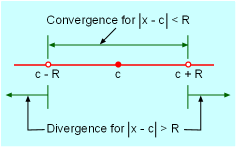Interval of Convergence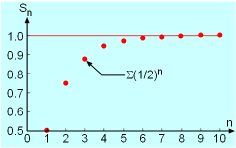Geometric Series Σxn For a given power series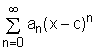, only three possibilities exist: (1) The power series converges only when x = c. (2) The power series converges for all x. (3) There is a number R > 0 such that the series converges absolutely for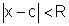, and the series diverges for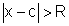, where R is the radius of convergence. The interval of convergence for a power series consists of all the values x of which the series is convergent. For example, the geometric series Σxn is a special case of the power series (an = 1 for all n and c = 0). It was shown previously that this series is convergent when -1 < x < 1 and it is divergent when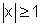. Hence, the radius of convergence is R = 1 and the interval of convergence is (-1, 1). The figure on the right shows the partial sum of the geometric series Σ(1/2)n, and it converges to 1. The following theorem is useful in differentiating and integrating a power series (term-by-term differentiation and integration): For a given power serieswith a radius of convergence R > 0, the function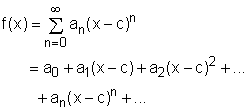is continuous and differentiable on the interval (a - R, a + R) and      (a)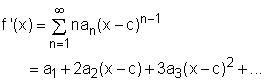(b)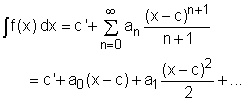Bessel Function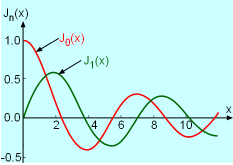Bessel Function of Order 0 and 1 Power series is useful and important mainly because it can be used to represent functions arise in mathematics, science and engineering applications. One such function is called the Bessel function. The Bessel function of order 0 is defined by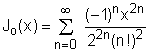while the Bessel function of order 1 is defined as: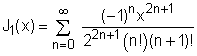Note that J'0(x) = - J1(x). The Bessel function appears when solving Kepler's equation for describing the motion of planets and when dealing with heat conduction problems. Bessel functions Jo(x) and J1(x) are plotted in the diagram on the left.